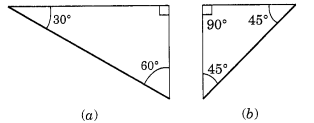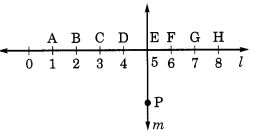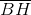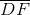## Ex 5.5 Question 1.

Which of the following are models for perpendicular lines:
(a) The adjacent edges of a table top.
(b) The lines of a railway track.
(c) The line segments forming a letter ‘L’.
(d) The letter V.
Solution:

(a) Yes, the adjacent edges of a tabletop are perpendicular to each other.
(b) No, the lines of railway tracks are parallel to each other.
(c) Yes, the line segments of ‘L’ are the model for perpendicular lines.
(d) No, the line segments of ‘V’ are not a model for perpendicular lines.

## Ex 5.5 Question 2.

Let$\overline { PQ }$  be perpendicular to the line segment$\overline { XY }$ . Let$\overline { PQ }$ and$\overline { XY }$ intersect at in the point A. What is the measure of ∠PAY?
Solution:

Since$\overline { PQ }$ ⊥ XY
∴ ∠PAY = 90°## Ex 5.5 Question 3.

There are two set-squares in your box. What are the measures of the angles that are formed at their corners? Do they have any angle measure that is common?
Solution:The measure of angles in fig. a = 300, 600 and 900

The measure of angles in fig. b = 450, 450 and 900

Yes, the angle of measure 900 is common in between them.

## Ex 5.5 Question 4.

Study the diagram. The line l is perpendicular to line m.(a) Is CE = EG?

(b) Does PE bisects CG?
(c) Identify any two line segments for which PE is the perpendicular bisector.
(d) Are these true?
(i) AC > FG
(ii) CD = GH
(iii) BC < EH
Solution:
(a) Yes, Since, CE = 2 units and EG = 2 units Hence, CE = EG.
(b)  Yes. Since, CE = EG as both is of 2 units. Hence PE bisect CG.
(c)and(d) (i) True (ii) True (iii) True

error: Content is protected !!